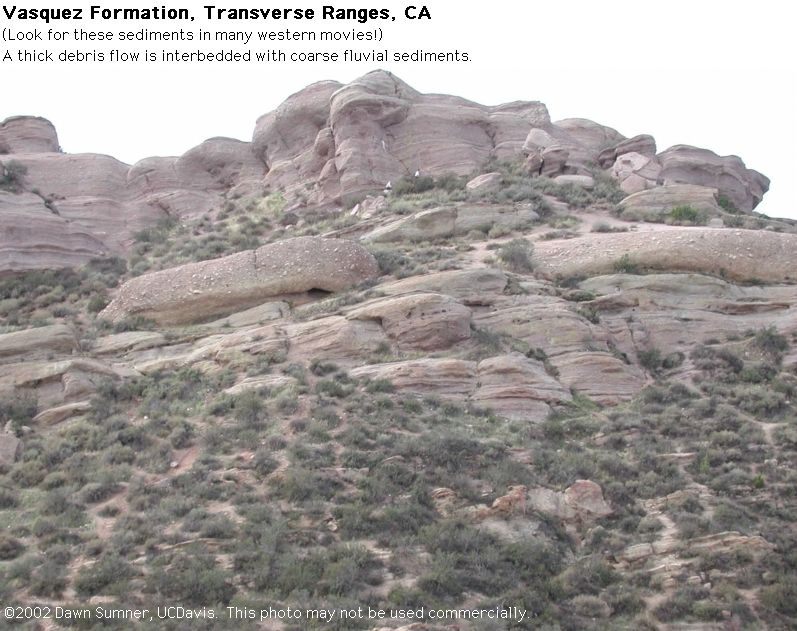# 6.1.4: Fluvial Deposits (Chapter 9)

$$\newcommand{\vecs}{\overset { \rightharpoonup} {\mathbf{#1}} }$$ $$\newcommand{\vecd}{\overset{-\!-\!\rightharpoonup}{\vphantom{a}\smash {#1}}}$$$$\newcommand{\id}{\mathrm{id}}$$ $$\newcommand{\Span}{\mathrm{span}}$$ $$\newcommand{\kernel}{\mathrm{null}\,}$$ $$\newcommand{\range}{\mathrm{range}\,}$$ $$\newcommand{\RealPart}{\mathrm{Re}}$$ $$\newcommand{\ImaginaryPart}{\mathrm{Im}}$$ $$\newcommand{\Argument}{\mathrm{Arg}}$$ $$\newcommand{\norm}{\| #1 \|}$$ $$\newcommand{\inner}{\langle #1, #2 \rangle}$$ $$\newcommand{\Span}{\mathrm{span}}$$ $$\newcommand{\id}{\mathrm{id}}$$ $$\newcommand{\Span}{\mathrm{span}}$$ $$\newcommand{\kernel}{\mathrm{null}\,}$$ $$\newcommand{\range}{\mathrm{range}\,}$$ $$\newcommand{\RealPart}{\mathrm{Re}}$$ $$\newcommand{\ImaginaryPart}{\mathrm{Im}}$$ $$\newcommand{\Argument}{\mathrm{Arg}}$$ $$\newcommand{\norm}{\| #1 \|}$$ $$\newcommand{\inner}{\langle #1, #2 \rangle}$$ $$\newcommand{\Span}{\mathrm{span}}$$$$\newcommand{\AA}{\unicode[.8,0]{x212B}}$$

# Fluvial Deposits at Vasquez Rocks County ParkFigure $$\PageIndex{1}$$: Graded bedding with faint cross stratification deposited in a fluvial channelFigure $$\PageIndex{2}$$: Debris Flow in a Fluvial EnvironmentFigure $$\PageIndex{3}$$: Gravel deposited in a channel​​​​​​​Figure $$\PageIndex{4}$$: Mud cracks that formed in an overbank deposit

# Fluvial Deposits Near Oberlin, OHFigure $$\PageIndex{5}$$: Quarry wall showing fluvial cross stratification, probably due to channel migration and bar accretion.​​​​​​​Figure $$\PageIndex{6}$$: Extension of the same quarry wall as above​​​​​​​Figure $$\PageIndex{7}$$: Large Cross Stratification​​​​​​​Figure $$\PageIndex{8}$$: Large Cross Stratification​​​​​​​Figure $$\PageIndex{9}$$: Cross stratified sediment deposited by a migrating bar based on the abundance of more planar laminae that would be characteristic of a channel bottom.Figure $$\PageIndex{10}$$: Different view of the bedform in the previous photoFigure $$\PageIndex{11}$$: Climbing dunes

# Ancient Fluvial Deposits near Lake Louise, Southern Canadian RockiesFigure $$\PageIndex{12}$$: Stratification from a migrating bar and subsequent channel fillFigure $$\PageIndex{13}$$: Stratification from a migrating bar​​​​​​​

The following photographs were taken by Jeff Mount during the Integrated Watershed Science class trip down the Kaskawulsh River, Yukon (funded by his Roy Schelman chair). This river was dammed by ice from the toe of a glacier, which created a very large lake. The ice dam broke catastrophically, producing a huge flood. The flood waters transported very large volumes of sediment in huge dunes. After normal river processes resumed, the river eroded into the flood deposits, exposing the cross stratification in these impressive dunes.Figure $$\PageIndex{14}$$: Lake shoreline terraces from the lake​​​​​​​Figure $$\PageIndex{15}$$: Lake shoreline terraces from the lake and cross stratification in the river bank. This cross stratification may have been deposited by the flood waters.Figure $$\PageIndex{16}$$: Remnants of giant dunes that migrated during the flood​​​​​​​Figure $$\PageIndex{17}$$: Large dune cross stratification and remnants of giant dunes​​​​​​​Figure $$\PageIndex{18}$$: Large dune cross stratification and remnants of giant dunes​​​​​​​